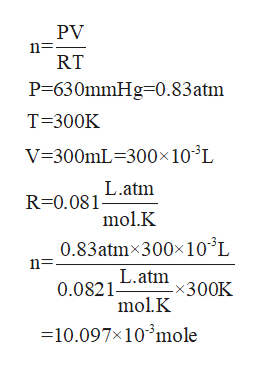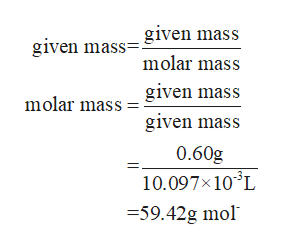# If 0.60 g of a gas occupies 300mL at 27°C and 630mm Hg of pressure, what is the molar mass of the gas?

Question
17 views

If 0.60 g of a gas occupies 300mL at 27°C and 630mm Hg of pressure, what is the molar mass of the gas?

check_circle

Step 1

Given that,

Mass of the gas=0.60 g

Volume of the gas=300mL

T=300K

P= 630mmHg

Step 2

We know that PV=nRT

Where n is the number of mole

Firstly you calculate the no. of molehelp_outlineImage TranscriptionclosePV n= RT P-630mmHg 0.83atm T 300K V 300mL 300x 103L L.atm R-0.081 molK 0.83atm 300x10'L L.atm 0.0821- x300K molK -10.097x103mole fullscreen
Step 3

Now you calculate the molar mass of th...help_outlineImage Transcriptionclosegiven mass given mass molar mass given mass molar mass given mass 0.60g 10.097x10 L =59.42g mol fullscreen

### Want to see the full answer?

See Solution

#### Want to see this answer and more?

Solutions are written by subject experts who are available 24/7. Questions are typically answered within 1 hour.*

See Solution
*Response times may vary by subject and question.
Tagged in

### Chemistry